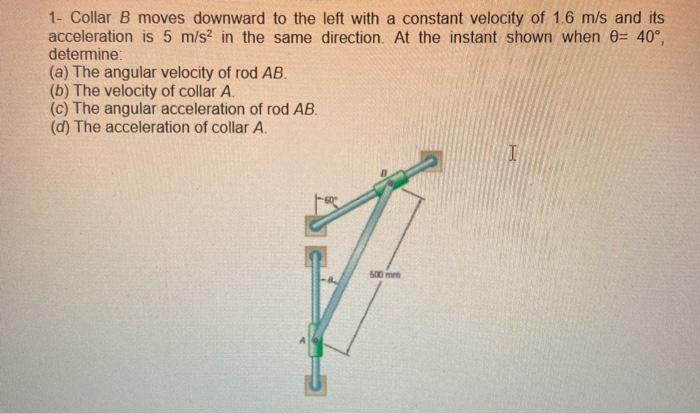# Question 1- Collar B moves downward to the left with a constant velocity of 1.6 m/s and its acceleration is 5 m/s2 in the same direction. At the instant shown when 0= 40°, determine: (a) The angular velocity of rod AB. (b) The velocity of collar A. (c) The angular acceleration of rod AB. (d) The acceleration of collar A. I 500 mmOAWBFH The Asker · Mechanical EngineeringTranscribed Image Text: 1- Collar B moves downward to the left with a constant velocity of 1.6 m/s and its acceleration is 5 m/s2 in the same direction. At the instant shown when 0= 40°, determine: (a) The angular velocity of rod AB. (b) The velocity of collar A. (c) The angular acceleration of rod AB. (d) The acceleration of collar A. I 500 mm
More
Transcribed Image Text: 1- Collar B moves downward to the left with a constant velocity of 1.6 m/s and its acceleration is 5 m/s2 in the same direction. At the instant shown when 0= 40°, determine: (a) The angular velocity of rod AB. (b) The velocity of collar A. (c) The angular acceleration of rod AB. (d) The acceleration of collar A. I 500 mm